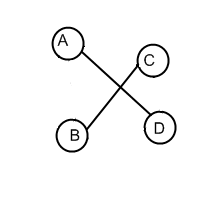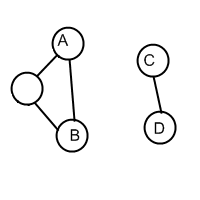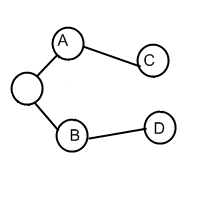### Problem Statement

There is a country with N cities, some of which are connected with bidirectional roads. Your task is to reconfigure the roads so that it is possible to get from each city to every other city. You must do this using the minimum possible number of transformations, where each transformation consists of the following steps:
1. Choose four different cities A, B, C and D, where roads (A, B) and (C, D) exist, but (A, C), (A, D), (B, C) and (B, D) do not exist.
2. Destroy roads (A, B) and (C, D).
3. Build two new roads - either (A, C) and (B, D), or (A, D) and (B, C).
The following images show an example of this transformation. From the first situation you can get the second one or the third one:You are given a String[] g, where the j-th character of the i-th element is 'Y' if there is a road between cities i and j, and 'N' otherwise. Return minimal number of transformations required to accomplish your task, or return -1 if it is impossible.

### Definition

 Class: StrangeCountry Method: transform Parameters: String[] Returns: int Method signature: int transform(String[] g) (be sure your method is public)

### Constraints

-g will contain between 2 and 50 elements, inclusive.
-Each element of g will contain exactly N characters 'Y' or 'N', where N is the number of elements in g.
-For each i and j, g[i][j] will be equal to g[j][i].
-For each i, g[i][i] will be equal to 'N'.

### Examples

0)

 ```{"NY", "YN"}```
`Returns: 0`
 This country is already connected.
1)

 ```{"NYYNN", "YNYNN", "YYNNN", "NNNNY", "NNNYN"}```
`Returns: 1`2)

 ```{"NYYNNNN", "YNYNNNN", "YYNNNNN", "NNNNYYN", "NNNYNYY", "NNNYYNY", "NNNNYYN"}```
`Returns: 1`
3)

 ```{"NYNYNNNNNNNN", "YNYNNNNNNNNN", "NYNYYNNNNNNN", "YNYNNNNNNNNN", "NNYNNYYNNNNN", "NNNNYNYNNNNN", "NNNNYYNNNNNN", "NNNNNNNNYYNN", "NNNNNNNYNYNN", "NNNNNNNYYNNN", "NNNNNNNNNNNY", "NNNNNNNNNNYN"}```
`Returns: 2`
4)

 ```{"NYNNNN", "YNYNNN", "NYNYNN", "NNYNNN", "NNNNNY", "NNNNYN"}```
`Returns: -1`

#### Problem url:

http://www.topcoder.com/stat?c=problem_statement&pm=10375

#### Problem stats url:

http://www.topcoder.com/tc?module=ProblemDetail&rd=13749&pm=10375

giolekva

#### Testers:

PabloGilberto , lovro , ivan_metelsky

#### Problem categories:

Graph Theory, Greedy